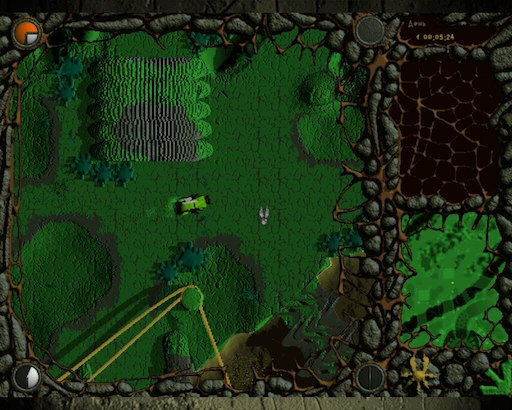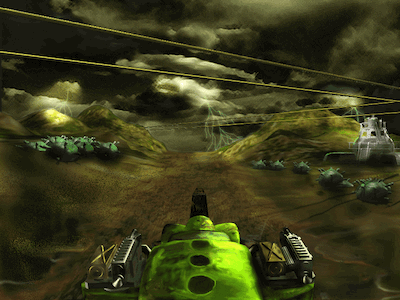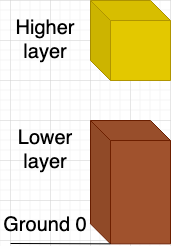Historically, Vangers terrain was rendered with a skewed top-down view:The data model, which we described in Data Formats, was designed for this view. Each point of the terrain was given 3 height values to encode the double-layer field. However, fans imagination often wondered about a possible universe, in which the mechos was seen from behind, or even from inside. This was fueled by one of the original loading screens:We’ve done a number of rendering experiments, each deserving a separate post, but never were we able to get as close to the real 3rd-person view as today with the “bar painting” technique.

## Algorithm

The core of the algorithm is drawing the input data in the most brute way possible. Each point of the map is represented as 2 vertical bars: one of the lower layer, one for the higher layer:### Geometry

Each bar has 5 quads, so we end up rendering 20 triangles per point (!) of the terrain, based on 16 vertices. This data makes up a rendering instance. All the terrain is drawn as a single instanced call, and the 2D region of visible surface is provided in uniforms. This allows the vertex shader to compute the bar position based on the instance index:

``````int row_size = int(ceil(u_SampleRange.y - u_SampleRange.x));
float rel_x = float(gl_InstanceIndex % row_size);
float rel_y = float(gl_InstanceIndex / row_size);
float x = u_SampleRange.x + rel_x;
float y = u_SampleRange.z + rel_y;
``````

Vertex positions relative to the current bar are computed in the vertex shader without any extra inputs:

``````Surface suf = get_surface(vec2(x, y));
float altitude = gl_VertexIndex >= 12 ? suf.high_alt :
gl_VertexIndex >= 8 ? suf.low_alt + suf.delta :
gl_VertexIndex >= 4 ? suf.low_alt : 0.0;
int cx = ((gl_VertexIndex + 0) & 0x3) >= 2 ? 1 : 0;
int cy = ((gl_VertexIndex + 1) & 0x3) >= 2 ? 1 : 0;
``````

The index data is written by hand and stored in a static index buffer on the GPU.

### Optimization

#### Visibility

One of the key optimizations to this algorithm is trying to minimize the number of points rendered in any given frame. Sending all the level at once, with its giant size of 16384x2048, is unfeasible.

To reduce the workload size, we compute a rough 2D axis-aligned subrectangle of the terrain by considering the camera frustum. We take the 4 endpoints as well as the origin of the camera, and build the 2D bound out of these 5 points:This is a rough approximation, but it allows our vertex shader to be fairly light, doesn’t require uploading any data (other than uniforms), and is easy to compute.

Note that it’s crucial to reduce the far plane of the camera for this approach to work. We had to add a simple fog effect to make the cutoff look more natural. The reasonable far plane value appeared to be around 500.

#### Ordering

Another very important optimization is ordering. We want to minimize the amount of overdraw when rendering terrain. For this to be the case, we need to ensure the bars appear on screen starting from the front and following into the back. Instead of collecting, sorting, and uploading the bar coordinates, we achieve this by simply tweaking the position generation algorithm in a way that takes camera orientation into consideration:

``````float x = u_CamOriginDir.z > 0.0 ? u_SampleRange.x + rel_x : u_SampleRange.y - rel_x;
float y = u_CamOriginDir.w > 0.0 ? u_SampleRange.z + rel_y : u_SampleRange.w - rel_y;
``````

With this tweak, XCode reports early Z test rejection to be 96%, which is great for us. It means, the main complexity of the algorithm is contained within vertex processing. Without the tweak, this number can be anything between 4% and 96%, and performance jumps around based on the camera orientation.

## Results

We have a large video uploaded to /r/rust_gamedev showing a one-minute ride with 3rd person camera. Here is another shot:Overall, given the listed optimizations and reduced far plane, this method is the only practical way we can get non-top-down cameras working in vange-rs. It’s fairly dumb in terms of used GPU features, performant enough, and produces exact rendering.

### Future work

Algorithm could be further optimized by doing a more careful collection of bars to process. A lot of terrain points are not double-layered, so the current approach wastes 50% of power on them. The visibility bounds can also be tightened more.

Drawing shadows using this method would be a big waste, especially since we want the light to be mostly above the surface. One of the other rendering techniques would work better, but we need to support this separation of rendering between the shadow and the main screen.